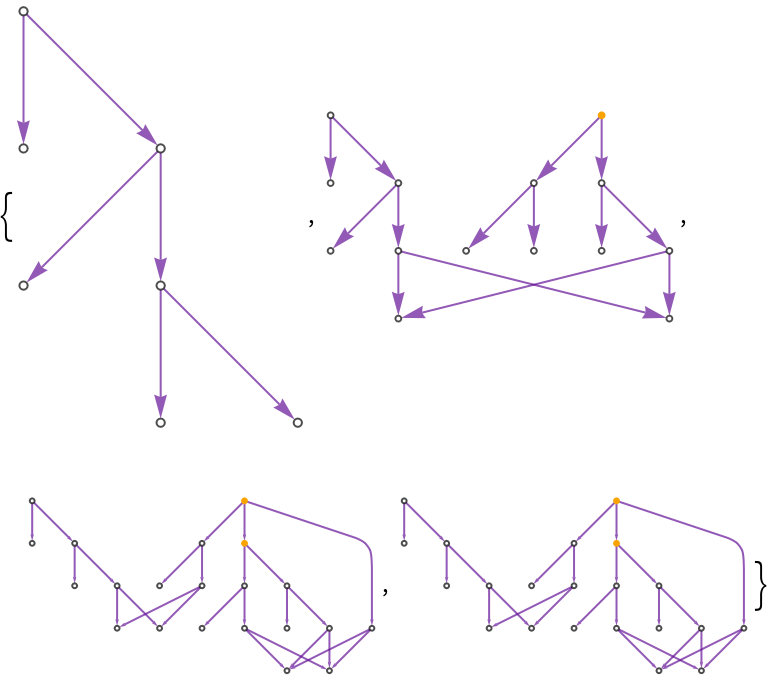#Function Repository Resource:

# CombinatorToDAG

Convert a combinator expression to a directed acyclic graph (DAG)

Contributed by: Wolfram Research
 ResourceFunction["CombinatorToDAG"][cmb] displays the combinator expression cmb as a directed acyclic graph, splitting the expression into heads and parts down to the level of atoms. ResourceFunction["CombinatorToDAG"][cmb,t] displays the possible subexpressions of the combinator expression cmb if cmb were allowed to evolve t steps, assuming the S and K combinator transformation rules. ResourceFunction["CombinatorToDAG"][rules,cmb,t] displays the possible subexpressions of the combinator expression cmb if cmb were allowed to evolve t steps via the possible transformations in the list rules.

## Details and Options

The input cmb must be given as a combinator expression in nested bracket form.
The combinator transformations of interest must be given in rules as a list of Rule or RuleDelayed elements.
The number of steps t should be a non-negative integer.
ResourceFunction["CombinatorToDAG"] accepts options from Graph, with the following additions:
 "SKGlyphs" {CombinatorS, CombinatorK} symbols used to specify default transformation rules "ShowCausalGraph" whether to display causal graph of combinator evolution

## Examples

### Basic Examples (3)

Display a combinator expression as a directed acyclic graph:

 In:=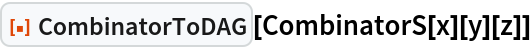Out=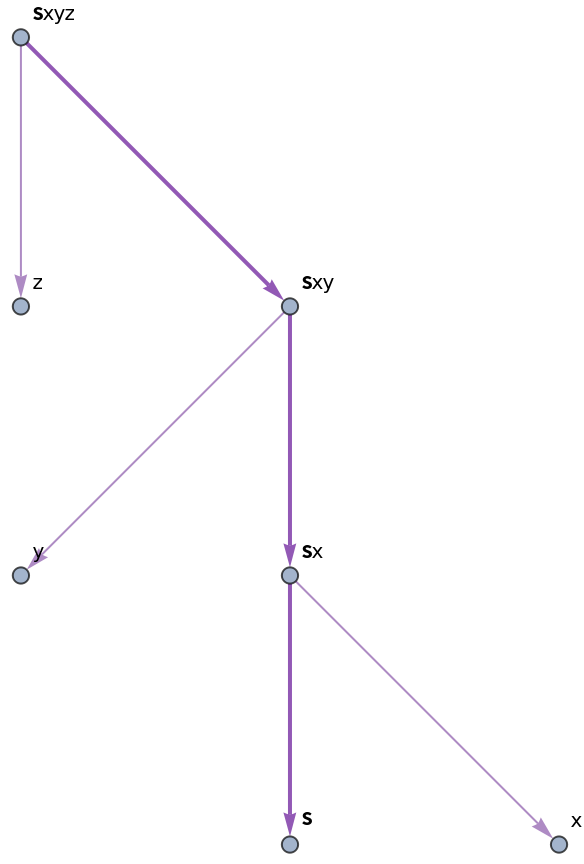Demonstrate how an initial combinator expression can be evolved over a single step:

 In:=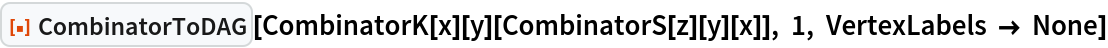Out=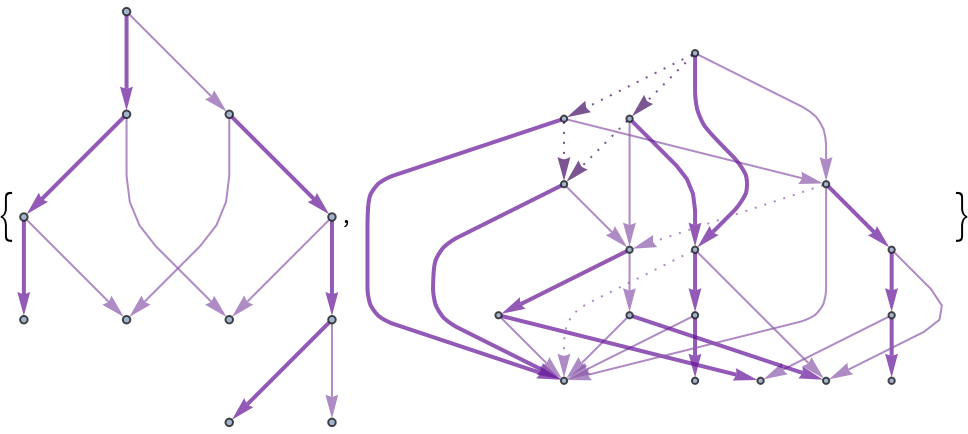Show the same DAGs, but this time labeled to clarify how the evolution works. A light purple dotted edge indicates direct application of a transformation rule, whereas a dark purple dotted line indicates application of a transformation rule to a subexpression:

 In:=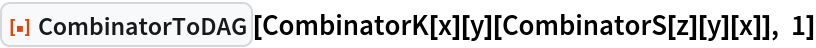Out=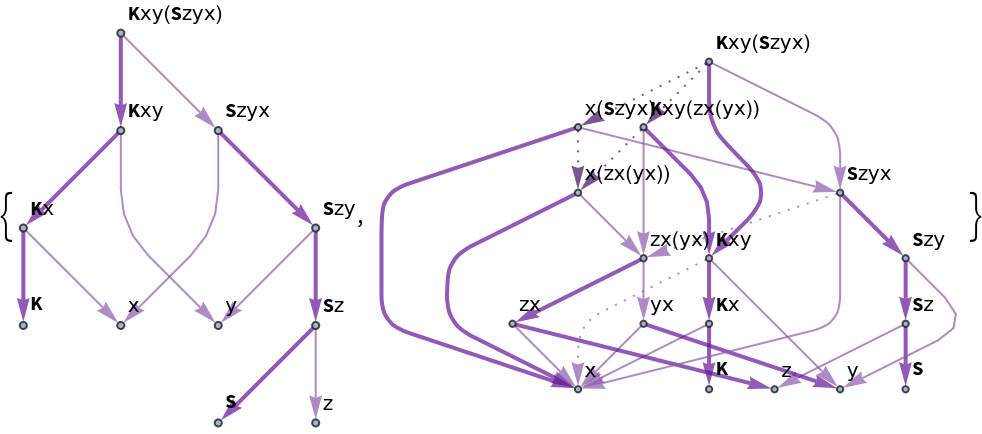Evolve a combinator expression over 2 steps using a specified rule:

 In:=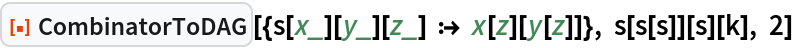Out=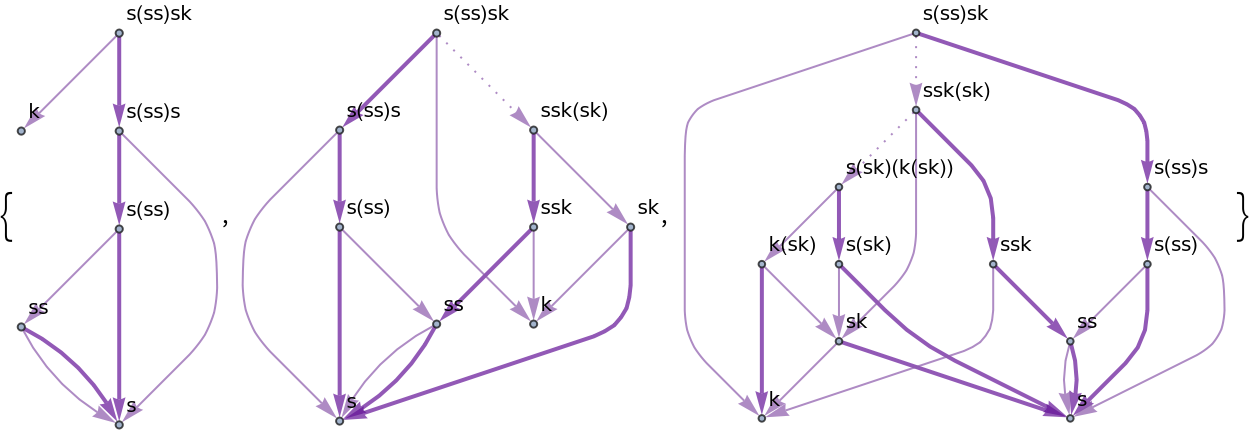### Options (3)

Evolve a combinator expression over 2 steps using the default rules with specified glyphs for the S and K combinators:

 In:=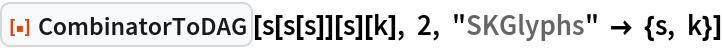Out=Display a causal graph of update events for a certain step of a combinator evolution (yellow nodes correspond to light purple arrows in the DAG representation, and gray nodes correspond to dark purple arrows):

 In:=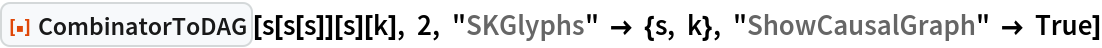Out=When a combinator expression reaches its fixed point (such that transformation rules can no longer be applied), the causal graph will cease to change:

 In:=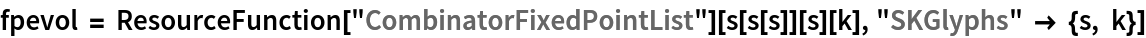Out=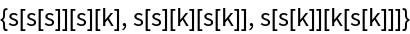In:=Out=